# Intermediate Algebra Tutorial 41

Intermediate Algebra
Tutorial 41: Rationalizing Denominators and Numerators

WTAMU > Virtual Math Lab > Intermediate AlgebraLearning Objectives

After completing this tutorial, you should be able to:
1. Rationalize one term denominators of rational expressions.
2. Rationalize one term numerators of rational expressions.
3. Rationalize two term denominators of rational expressions.Introduction

In this tutorial we will talk about rationalizing the denominator and numerator of rational expressions.  Recall from Tutorial 3: Sets of Numbers that a rational number is a number that can be written as one integer over another. Recall from Tutorial 3: Sets of Numbers that an irrational number is not one that is hard to reason with but is a number that cannot be written as one integer over another.  It is a non-repeating, non-terminating decimal. One example of an irrational number is when you have a root of an expression that is not a perfect root, for example, the square root of 7 or the cube root of 2.  So when we rationalize either the denominator or numerator we want to rid it of radicals.Tutorial

Rationalizing the Denominator
(with one term)

When a radical contains an expression that is not a perfect root, for example, the square root of 3 or cube root of 5,  it is called an irrational number.  So, in order to rationalize the denominator, we need to get rid of all radicals that are in the denominator.

Step 1: Multiply numerator and denominator by a radical that will get rid of the radical in the denominator.

If the radical in the denominator is a square root, then you multiply by a square root that will give you a perfect square under the radical when multiplied by the denominator.   If the radical in the denominator is a cube root, then you multiply by a cube root that will give you a perfect cube under the radical when multiplied by the denominator and so forth...

Note that the phrase "perfect square" means that you can take the square root of it.  Just as "perfect cube" means we can take the cube root of the number, and so forth.

Keep in mind that as long as you multiply the numerator and denominator by the exact same thing, the fractions will be equivalent.

Step 2: Make sure all radicals are simplified.

Some radicals will already be in a simplified form, but make sure you simplify the ones that are not.  If you need a review on this, go to Tutorial 39: Simplifying Radical Expressions.

Step 3: Simplify the fraction if needed.

Be careful.  You cannot cancel out a factor that is on the outside of a radical with one that is on the inside of the radical.  In order to cancel out common factors, they have to be both inside the same radical or be both outside the radical.Example 1:   Rationalize the denominator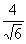.

Step 1: Multiply numerator and denominator by a radical that will get rid of the radical in the denominator.

Since we have a square root in the denominator, then we need to multiply by the square root of an expression that will give us a perfect square under the radical in the denominator.

Square roots are nice to work with in this type of problem because if the radicand is not a perfect square to begin with, we just have to multiply it by itself and then we have a perfect square.

So in this case we can accomplish this by multiplying top and bottom by the square root of 6: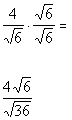*Mult. num. and den. by sq. root of 6

*Den. now has a perfect square under sq. root

AND

Step 3: Simplify the fraction if needed.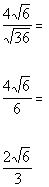*Sq. root of 36 is 6

*Divide out the common factor of 2

Be careful when you reduce a fraction like this.  It is real tempting to cancel the 3 which is on the outside of the radical with the 6 which is inside the radical on the last fraction.  You cannot do that unless they are both inside the same radical or both outside the radical like the 4 in the numerator and the 6 in the denominator were in the second to the last fraction.Example 2:   Rationalize the denominator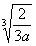.

Step 1: Multiply numerator and denominator by a radical that will get rid of the radical in the denominator.

Since we have a cube root in the denominator, we need to multiply by the cube root of an expression that will give us a perfect cube under the radical in the denominator.

So in this case, we can accomplish this by multiplying top and bottom by the cube root of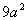: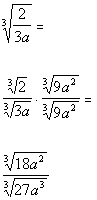*Mult. num. and den. by cube root of*Den. now has a perfect cube under cube root

AND

Step 3: Simplify the fraction if needed.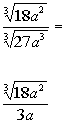*Cube root of 27 a cube is 3a

As discussed in example 1, we would not be able to cancel out the 3 with the 18 in our final fraction because the 3 is on the outside of the radical and the 18 is on the inside of the radical.

Also, we cannot take the cube root of anything under the radical.  So, the answer we have is as simplified as we can get it.

Rationalizing the Numerator
(with one term)

As mentioned above, when a radical cannot be evaluated,  for example, the square root of 3 or cube root of 5,  it is called an irrational number.  So, in order to rationalize the numerator, we need to get rid of all radicals that are in the numerator.

Note that these are the same basic steps for rationalizing a denominator, we are just applying to the numerator now.

Step 1: Multiply numerator and denominator by a radical that will get rid of the radical in the numerator.

If the radical in the numerator is a square root, then you multiply by a square root that will give you a perfect square under the radical when multiplied by the numerator.   If the radical in the numerator is a cube root, then you multiply by a cube root that will give you a perfect cube under the radical when multiplied by the numerator and so forth...

Note that the phrase "perfect square" means that you can take the square root of it.  Just as "perfect cube" means we can take the cube root of the number, and so forth.

Keep in mind that as long as you multiply the numerator and denominator by the exact same thing, the fractions will be equivalent.

Step 2: Make sure all radicals are simplified.

Some radicals will already be in a simplified form, but make sure you simplify the ones that are not.  If you need a review on this, go to Tutorial 39: Simplifying Radical Expressions.

Step 3: Simplify the fraction if needed.

Be careful.  You cannot cancel out a factor that is on the outside of a radical with one that is on the inside of the radical.  In order to cancel out common factors, they have to be both inside the same radical or be both outside the radical.Example 3:   Rationalize the numerator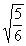.

Step 1: Multiply numerator and denominator by a radical that will get rid of the radical in the numerator.

Since we have a square root in the numerator, then we need to multiply by the square root of an expression that will give us a perfect square under the radical in the numerator.

So in this case, we can accomplish this by multiplying top and bottom by the square root of 5: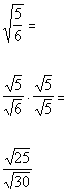*Mult. num. and den. by sq. root of 5

*Num. now has a perfect square under sq. root

AND

Step 3: Simplify the fraction if needed.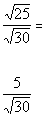*Sq. root of 25 is 5

As discussed above, we would not be able to cancel out the 5 with the 30 in our final fraction because the 5 is on the outside of the radical and the 30 is on the inside of the radical.

Also, we cannot take the square root of anything under the radical.  So, the answer we have is as simplified as we can get it.Example 4:   Rationalize the numerator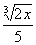.

Step 1: Multiply numerator and denominator by a radical that will get rid of the radical in the numerator.

Since we have a cube root in the numerator,  we need to multiply by the cube root of an expression that will give us a perfect cube under the radical in the numerator.

So in this case, we can accomplish this by multiplying top and bottom by the cube root of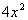: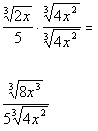*Mult. num. and den. by cube root of*Num. now has a perfect cube under cube root

AND

Step 3: Simplify the fraction if needed.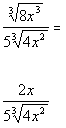*Cube root of 8 x cube is 2x

As discussed above, we would not be able to cancel out the 2x with the 4 x squared in our final fraction, because the 2x is on the outside of the radical and the 4 x squared is on the inside of the radical.

Also, we cannot take the cube root of anything under the radical.  So, the answer we have is as simplified as we can get it.

Rationalizing the Denominator
(with two terms)

Above we talked about rationalizing the denominator with one term.  Again, rationalizing the denominator means to get rid of any radicals in the denominator.

Because we now have two terms, we are going to have to approach it differently than when we had one term, but the goal is still the same.

Step 1: Find the conjugate of the denominator.

You find the conjugate of a binomial by changing the sign that is between the two terms, but keep the same order of the terms.

a + b and a - b are conjugates of each other.

Step 2: Multiply the numerator and the denominator of the fraction by the conjugate found in Step 1 .

Keep in mind that as long as you multiply the numerator and denominator by the exact same thing, the fractions will be equivalent.

Step 3: Make sure all radicals are simplified.

Some radicals will already be in a simplified form, but make sure you simplify the ones that are not.  If you need a review on this, go to Tutorial 39: Simplifying Radical Expressions.

Step 4: Simplify the fraction if needed.

Be careful.  You cannot cancel out a factor that is on the outside of a radical with one that is on the inside of the radical.  In order to cancel out common factors, they have to be both inside the same radical or be both outside the radical.Example 5:   Rationalize the denominator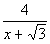Step 1: Find the conjugate of the denominator.

In general the conjugate of a + b is a - b and vice versa.

So what would the conjugate of our denominator be?

It looks like the conjugate is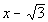.

Step 2: Multiply the numerator and the denominator of the fraction by the conjugate found in Step 1.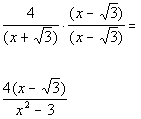*Mult. num. and den. by conjugate of den.
*Product of the sum and diff. of two terms

AND

Step 4: Simplify the fraction if needed.

No simplifying can be done on this problem so the final answer is: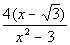Example 6:   Rationalize the denominator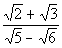.

Step 1: Find the conjugate of the denominator.

In general the conjugate of a + b is a - b and vice versa.

So what would the conjugate of our denominator be?

It looks like the conjugate is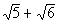.

Step 2: Multiply the numerator and the denominator of the fraction by the conjugate found in Step 1 .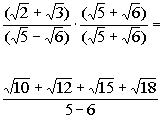*Mult. num. and den. by conjugate of den.
*Use FOIL method in num.
*Product of the sum and diff. of two terms

AND

Step 4: Simplify the fraction if needed.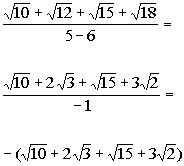*12 is (4)(3) and sq. root of 4 is 2
*18 is (9)(2) and sq. root of 9 is 3Practice Problems

These are practice problems to help bring you to the next level.  It will allow you to check and see if you have an understanding of these types of problems. Math works just like anything else, if you want to get good at it, then you need to practice it.  Even the best athletes and musicians had help along the way and lots of practice, practice, practice, to get good at their sport or instrument.  In fact there is no such thing as too much practice.

To get the most out of these, you should work the problem out on your own and then check your answer by clicking on the link for the answer/discussion for that  problem.  At the link you will find the answer as well as any steps that went into finding that answer.Practice Problem 1a: Rationalize the Denominator.

1a.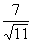Practice Problem 2a: Rationalize the Numerator.

2a.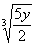Practice Problem 3a: Rationalize the Denominator.

3a.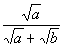Need Extra Help on these Topics?

Go to Get Help Outside the Classroom found in Tutorial 1: How to Succeed in a Math Class for some more suggestions.Last revised on July 21, 2011 by Kim Seward.